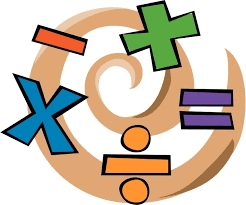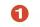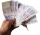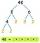# Most divisors

From the natural numbers from 1 to 100, find the one that has the most divisors.

x =  60
y =  72

### Step-by-step explanation:Did you find an error or inaccuracy? Feel free to write us. Thank you!Tips to related online calculators
Do you want to perform natural numbers division - find the quotient and remainder?

## Related math problems and questions:

• Prime divisorsFind 2/3 of the sum's ratio and the product of all prime divisors of the number 120.
• DivisorsFind all divisors of number 493. How many are them?
• No. of divisorsHow many different divisors have number 13 4 * 2 4?
• Common divisorsFind all common divisors of numbers 30 and 45.
• Reminder and quotientThere are given the number C = 281, D = 201. Find the highest natural number S so that the C:S, D:S are with the remainder of 1,
• DivisibilityDetermine all divisors of number 84.
• Reminder and quotientThere are given numbers A = 135, B = 315. Find the smallest natural number R greater than 1 so that the proportions R:A, R:B are with the remainder 1.
• Game roomWinner can took win in three types of jettons with value 3, 30 and 100 dollars. What is minimal value of win payable in this values of jettons?
• DivisorsThe sum of all divisors unknown odd number is 2112. Determine sum of all divisors of number which is twice of unknown numbers.
• BanknotesHow many different ways can the cashier payout € 310 if he uses only 50 and 20 euro banknotes? Find all solutions.
• AthletesAthletes at the stadium could enter two-steps, three-steps, four-steps, five-steps, six-steps. There were more than 100 but less than 200. How many athletes were there?
• DecompositionMake decomposition using prime numbers of number 155. Result write as prime factors (all, even multiple)
• DivisibilityWrite all the integers x divisible by seven and eight simultaneously, for which the following applies: 100 < x < 200.
• 7 digit numberIf 3c54d10 is divisible by 330, what is the sum of c and d?
• Find the sumFind the sum of all natural numbers from 1 and 100, which are divisible by 2 or 5
• The HotelThe Holiday Hotel has the same number of rooms on each floor. Rooms are numbered with natural numerals sequentially from the first floor, no number is omitted, and each room has a different number. Three tourists arrived at the hotel. The first one was in
• Amazing numberAn amazing number is name for such even number, the decomposition product of prime numbers has exactly three not necessarily different factors and the sum of all its divisors is equal to twice that number. Find all amazing numbers.